on 25-May-2016 (Wed)

Flashcard 1345820691724

Tags
#scientific_view_of_the_world
Question
science depends on a transmission of [...]
ideas

status measured difficulty not learned 37% [default] 0

pdf

cannot see any pdfs

Annotation 1346351271180

 In mathematics, an isomorphism (from the Ancient Greek: ἴσος isos "equal", and μορφή morphe "form" or "shape") is a homomorphism or morphism (i.e. a mathematical mapping) that admits an inverse.[note 1] Two mathematical objects are isomorphic if an isomorphism exists between them. An automorphism is an isomorphism whose source and target coincide. The interest of isomorphisms lies in the fact that two isomorphic objects cannot be distinguished by using only the properties used to define morphisms; thus isomorphic objects may be considered the same as long as one considers only these properties and their consequences. For most algebraic structures, including groups and rings, a homomorphism is an isomorphism if and only if it is bijective. In topology, where the morphisms are continuous functions, isomorphisms are also called homeomorphisms or bicontinuous functions. In mathematical analysis, where the morphisms are differentiable functions, isomorphisms are also called diffeomorphisms. A canonical isomorphism is a canonical map that is an isomorphism. Two objects are said to be canonically isomorphic if there is a canonical isomorphism between them. For example, the canonical map from a finite-dimensional vector space V to its second dual space is a canonical isomorphism; on the other hand, V is isomorphic to its dual space but not canonically in general. Isomorphisms are formalized using category theory. A morphism f : X → Y in a category is an isomorphism if it admits a two-sided inverse, meaning that there is another morphism g : Y → X in that category such that gf = 1X and fg = 1Y , where 1X and 1Y...

Isomorphism - Wikipedia, the free encyclopedia
s article is about mathematics. For other uses, see Isomorphism (disambiguation). The group of fifth roots of unity under multiplication is isomorphic to the group of rotations of the regular pentagon under composition. <span>In mathematics, an isomorphism (from the Ancient Greek: ἴσος isos "equal", and μορφή morphe "form" or "shape") is a homomorphism or morphism (i.e. a mathematical mapping) that admits an inverse. [note 1] Two mathematical objects are isomorphic if an isomorphism exists between them. An automorphism is an isomorphism whose source and target coincide. The interest of isomorphisms lies in the fact that two isomorphic objects cannot be distinguished by using only the properties used to define morphisms; thus isomorphic objects may be considered the same as long as one considers only these properties and their consequences. For most algebraic structures, including groups and rings, a homomorphism is an isomorphism if and only if it is bijective. In topology, where the morphisms are continuous functions, isomorphisms are also called homeomorphisms or bicontinuous functions. In mathematical analysis, where the morphisms are differentiable functions, isomorphisms are also called diffeomorphisms. A canonical isomorphism is a canonical map that is an isomorphism. Two objects are said to be canonically isomorphic if there is a canonical isomorphism between them. For example, the canonical map from a finite-dimensional vector space V to its second dual space is a canonical isomorphism; on the other hand, V is isomorphic to its dual space but not canonically in general. Isomorphisms are formalized using category theory. A morphism f : X → Y in a category is an isomorphism if it admits a two-sided inverse, meaning that there is another morphism g : Y → X in that category such that gf = 1 X and fg = 1 Y , where 1 X and 1 Y are the identity morphisms of X and Y, respectively.  Contents 1 Examples 1.1 Logarithm and exponential 1.2 Integers modulo 6 1.3 Relation-preserving isomorphism 2 Isomorphism vs. bijective morphism 3 Applications 4 Relation with equ

#has-images

Logarithm and exponential [ edit ]

Let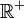be the multiplicative group of positive real numbers, and let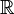be the additive group of real numbers.

The logarithm function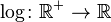satisfies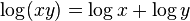for all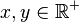, so it is a group homomorphism. The exponential function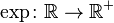satisfies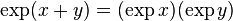for all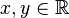, so it too is a homomorphism.

The identities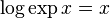and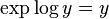show that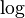and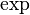are inverses of each other. Sinceis a homomorphism that has an inverse that is also a homomorphism,is an isomorphism of groups.

Becauseis an isomorphism,

...